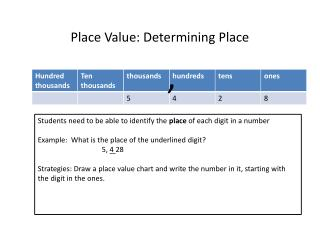DownloadDownload PresentationPlace Value: Determining Place

# Place Value: Determining Place

Download Presentation## Place Value: Determining Place

- - - - - - - - - - - - - - - - - - - - - - - - - - - E N D - - - - - - - - - - - - - - - - - - - - - - - - - - -
##### Presentation Transcript

1. Place Value: Determining Place , Students need to be able to identify the place of each digit in a number Example: What is the place of the underlined digit? 5, 4 28 Strategies: Draw a place value chart and write the number in it, starting with the digit in the ones.

2. Place Value: Determining Value Steps: Write the underlined digit. Count the number of places after the underlined digit. Write that many zeros. Example: 5,428 1. Write the 4. 2. There are 2 places (the tens and ones) after the 4. 3. Write two zeros after the 4. 4. The answer is 400.

3. Comparing Numbers 5,367 5,639 Strategy: 1. Write the numbers so one is below the other. 5,367 5,639 2. Look at the first digits of each number. If they are different, you can figure out the number that is greatest. 3. If they are the same, you must look at the next place. In the example, the digits in the thousands place are the same so we must look at the hundreds place. The 6 is greater than the 3 so 5,639 > 5,367. Remember the ‘mouth’ points to the greater number.

4. Ordering Numbers 3,589 4,789 567 3,501 Strategy: It is easier to compare the numbers if you stack them one on top of the other. 3,589 4,789 567 3,501 Look at the first digit to determine the greatest number. If the 2 digits are the same, you must look at the digit in the next place. In the example, the 4 is greatest so 4,789 is the greatest number. 3,589 4,789 4,789 3,589 567 3,501 3,501 567 The next 2 numbers have a 3 in the thousands place so we need to look at the hundreds place. Both have a 5 so we need to look at the tens place. The 8 is greater than 0 so 3,589 is greater than 3,501.

5. Rounding Steps: 1. Circle the digit that is in the place you are rounding to. 2. Underline the digit just to the right. This is your “bossy neighbor” number. 3. The “bossy neighbor” tells us whether to round down or round up. Rule: If the “bossy neighbor” is a 0,1,2,3 or 4, round down. If the “bossy neighbor” is a 5,6,7,8 or 9 round up. ___________________________________________________________ Example 1. Round 634 to the nearest hundred. 634 600 700 The 3 tells us to round down to 600.

6. Example 2: Round 3,789 to the nearest thousand. 3,789 3,000 4,000 The 7 tells us to round up to 4,000. Example 3: Round 217 to the nearest ten. 217 210 220 Don’t forget to write the digits that are before the underlined digit. The 7 tells us to round up to 220.

7. Writing Numbers 6,728 Standard Form: 6, 728 Expanded Form: 6,000 + 700 + 20 + 8 Word Form: six thousand, seven hundred twenty-eight Hints: Say the number in your head. Say ‘thousand’ at the comma (,).